# RS Aggarwal Class 10 Solutions Chapter 4 - Triangles Ex 4C(4.3)

## RS Aggarwal Class 10 Chapter 4 - Triangles Ex 4C(4.3) Solutions Free PDF

Students of Class 10 should understand the importance of solutions provided here to get a deep knowledge of the subject and from the competitive exam point of view. It will help students to score well in their examinations clearing all their doubts and concepts related to any of the topics. The RS Aggarwal Class 10 solutions is an all in one need for every student who faces difficulties and have doubts in mathematics.

We at BYJU’S provide the students with in-depth appropriate step-by-step solutions to clarify their doubts and boost their overall academic performance. Practicing these solutions help these students to know the best effective way to answer questions in the exams so that they can score really well. All the RS Aggarwal Solutions Class 10 Chapter 4 Triangles Ex 4C will help you in doing quick revision and perform well in the exam.

## Download PDF of RS Aggarwal Class 10 Solutions Chapter 4 – Triangles Ex 4C (4.3)

Question 1: ABC is an isosceles triangle, right-angled at B. Similar triangles ACD and ABE are constructed on sides AC and AB. Find the ratio between the areas of $\Delta$ABE and $\Delta$ACD.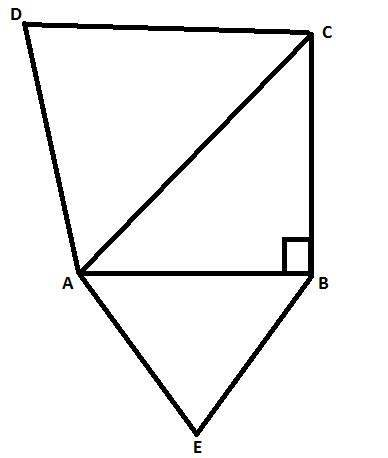Solution:

ABC is an isosceles triangle right angled at B,

Let AB = BC = x cm

By Pythagoras theorem,

AC2 = AB2 + BC2 = x2 + x2

AC2 = 2x2

AC = $\sqrt{2}$x

$\Delta$ACD $\approx$ $\Delta$ABE (Given)

Therefore, $\frac{ar\Delta ABE}{ar\Delta ACD}=\frac{AB^{2}}{AC^{2}}=\frac{x^{2}}{(\sqrt{2}x)^{2}}=\frac{x^{2}}{2x^{2}}=\frac{1}{2}=1:2$

Question 2: An airplane leaves an airport and flies due north at a speed of 1000 km per hour. At the same time, another airplane leaves the same airport and flies due west at a speed of 1200 km per hour. How far apart will be the two planes after 1 ½ hours?

Solution: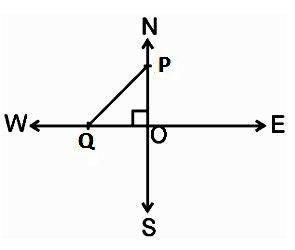Speed of the first aeroplane = 1000 km/hr

Distance covered by first aeroplane due north in $1\frac{1}{2}$ hours

(OA) = 1000 x 3/2 km = 1500 km

Sped of the second aeroplane = 1200 km/hr

Distance covered by second aeroplane due west in $1\frac{1}{2}$ hours

(OB) = 1200 x 3/2 km = 1800 km

In right angle ΔPOQ, we have

PQ2 = PO2 + OQ2

⇒ PQ2 = (1500)2 + (1800)2

⇒ PQ = $\sqrt{2250000+3240000}$ = $\sqrt{5490000}$ = $300\sqrt{61}$ km

Hence, the distance between two aeroplanes will be $300\sqrt{61}$ km.

Question 3: In a $\Delta$ABC, AD is a median and AL $\perp$ BC.

Prove that:

(a)AC2 = AD2 + BC.DL + $\left ( \frac{BC}{2} \right )^{2}$

(b) AB2 = AD2 – BC.DL + $\left ( \frac{BC}{2} \right )^{2}$

(c) AC2 + AB2 = 2AD2 + ½ BC2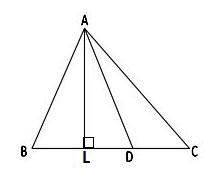Solution:

(a) In right triangle ALD

Using pythagoras theorem, we have

$AL^{2} = AD^{2} – DL^{2}$…….(i)

Again, in right triangle ACL

Using pythagoras theorem, we have

$AC^{2} = AL^{2} + LC^{2}$

$= AD^{2} – DL^{2} +(DL +DC)^{2}$ [ Using (i) ]

$= AD^{2} – DL^{2} + (DL + \frac{BC}{2})^{2}$  [  $Since, AD$ is a median ]

$= AD^{2} – DL^{2} + DL ^{2} + \left ( \frac{BC}{2} \right )^{2} + BC.DL$

$Therefore, AC^{2} = AD^{2} + BC.DL + \left ( \frac{BC}{2} \right )^{2}$ ………(ii)

(b) In right triangle ALD

Using pythagoras theorem, we have

$AL^{2} = AD^{2} – DL^{2}$…..(iii)

Again, in right triangle ABL

Using pythagoras theorem, we have

$AB^{2} = AL^{2} + LB^{2}$

$= AD^{2} – DL^{2} + LB^{2}$[ Using (iii) ]

$= AD^{2} – DL^{2} +(BD – DL)^{2}$

$= AD^{2} – DL^{2} + ( \frac{1}{2} BC – DL )^{2}$

$= AD^{2} – DL^{2} + \left ( \frac{BC}{2} \right )^{2} – BC.DL + DL^{2}$

$Therefore, AB^{2} = AD^{2} + BC.DL + \left ( \frac{BC}{2} \right )^{2}$  ………(iv)

(c) Adding (ii) and (iv) we have

$AC^{2} + AB^{2} = AD^{2} +BC.DL + \left ( \frac{BC}{2} \right )^{2} + AD^{2} – BC.DL + \left ( \frac{BC}{2} \right )^{2}$

$= 2 AD^{2} + \frac{BC^{2}}{4} + \frac{BC^{2}}{4}$

$= 2 AD^{2} + \frac{1}{2} BC^{2}$

Question 22: Naman is doing fly-fishing in a stream. The tip of his fishing rod is 1.8 m above the surface of the water and the fly at the end of the string rests on the water 3.6 m away from him and 2.4 m from the point directly under the tip of the rod. Assuming that the string (from the tip of his rod to the fly) is taut, how much string does he have out (see the adjoining figure)? If he pulls in the string at the rate of 5 cm per second, what will be the horizontal distance of the fly from him after 12 seconds?

Solution: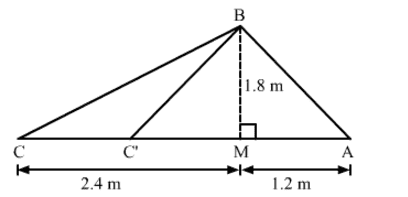Naman pulls in the string at the rate of 5 cm per second.

Hence, after 12 second the length of the string he will pull is given by

$12 \times 5 = 60$ cm or 0.6 m

By using Pythagoras theorem, we have

$BC^{2} = CM^{2} + MB^{2}$

$= (2.4)^{2} + (1.8)^{2}$ = 9

$Therefore, BC = 3$ m

${BC}’ = BC – 0.6$ = 3 – 0.6 = 2.4 m

Now, in $\bigtriangleup B{C}’M$

By using Pythagoras theorem, we have

${C}’M^{2} = B{C}’^{2} – MB^{2}$

$= (2.4)^{2} – (1.8)^{2}$ = 2.52

$Therefore, {C}’M = 1.6$ m

The horizontal distance of the fly from him after 12 seconds is given by

${C}’A = {C}’M + MA$ = 1.6 + 1.2 = 2.8 m

## EXERCISE 4 E

Q1: State the two properties which are necessary for given two triangles to be similar

Sol:

Two triangles are similar if and only if

1. The corresponding sides are in proportion.

2. The corresponding angles are equal.

Q2: State the basic proportionality theorem.

Sol:

If a line is drawn parallel to one side of a triangle intersect the other two sides, then it divides the other two sides in the same ratio.

Q3: State the converse of Thales theorem.

Sol:

If a line divides any two sides of a triangle in the same ratio, then the line must be parallel to the third side.

Q4: State the midpoint theorem.

Sol:

The line segment connecting the midpoints of two sides of a triangle is parallel to the third side and is equal to one half of the third side.

Q5: State the AAA- similarity criterion.

Sol:

If the corresponding angles of two triangles are equal, then their corresponding sides are proportional and hence the triangles are similar.

Q6: State the AA- similarity criterion.

Sol:

If two angles of a triangle are correspondingly equal to the two angles of another triangle, then the two triangles are similar.

Q7: State the SSS-criterion for the similarity of triangles.

Sol:

If the corresponding sides of two triangles are proportional then their corresponding angles are equal, and hence the two triangles are similar.

Q8: State the SAS- similarity criterion.

Sol:

If one angle of a triangle is equal to one angle of the other triangle and the sides including these angles are proportional then the two triangles are similar.

Q9: State Pythagoras‘ theorem.

Sol:

The square of the hypotenuse is equal to the sum of the square of the other two sides. Here, the hypotenuse is the longest side and it’s always, opposite to the right angle.

Q10: State the converse of Pythagoras’ theorem.

Sol:

If the square of one side of a triangle is equal to the sum if the squares of the other two sides, then the triangle is a right triangle.

Q11: If D,E and F are respectively the midpoints of sides AB,BC and CA of $\bigtriangleup ABC$, then what is the ratio of the areasof $\bigtriangleup DEF$ and $\bigtriangleup ABC$

Sol: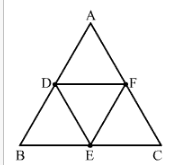By using mid-point theorem i.e. the segment joining two sides of a triangle at the midpoint of those sides is parallel to the third side and is half to the third side and is half the length of the third side.

$Therefore, DF \parallel BC$

And

$Therefore, DF = \frac{1}{2}BC$

$\Rightarrow DF = \frac{1}{2}BE$

Since, the opposite sides of the quadrilateral are parallel and equal.

Hence, BDFE is a parallelogram.

Similarly, DFCE is a parallelogram.

Now, In $\bigtriangleup BAC \;\; and \;\; \bigtriangleup EFD$

$\angle ABC = \angle EFD$ (Opposite angles of a parallelogram )

$\angle BCA = \angle EDF$ (Opposite angles of a parallelogram )

By AA similarity criterion, $\bigtriangleup ABC \sim \bigtriangleup EFD$

If two triangle are similar, then the ratio of their areas is equal to the ratio of the squares of their corresponding sides.

$Therefore, \frac{area (\bigtriangleup DEF)}{area (\bigtriangleup ABC)} =\left ( \frac{DF}{BC} \right )^{2} = \left ( \frac{DF}{2.DF} \right )^{2} = \frac{1}{4}$

Hence, the ratio of the areas of $\bigtriangleup DEF \;\; and \;\; \bigtriangleup ABC$ is 1:4.

Q.12: Two triangles ABC and PQR are such that AB = 3 cm, AC= 6 cm, $\angle A = 70^{\circ}, \; PR = 9 \; cm, \; \angle P = 70^{\circ} \;\; and \;\; PQ = 4.5 \; cm$. Show that $\bigtriangleup ABC \sim \bigtriangleup PQR$ and state the similarity theorem.

Sol:

Now, In $\bigtriangleup ABC \;\; and \;\; \bigtriangleup PQR$

$\angle A = \angle P = 70^{\circ}$

$\frac{AB}{PQ} = \frac{AC}{PR} \left [ Since, \frac{3}{4.5} = \frac{6}{9} \Rightarrow \frac{1}{1.5} = \frac{1}{1.5}\right ]$

By SAS similarity criterion, $\bigtriangleup ABC \sim \bigtriangleup PQR$

Q.13: If $\bigtriangleup ABC \sim \bigtriangleup DEF$ such that $2AB = DE \;\; and \;\; BC = 6 \; cm$, find EF.

Sol:

When two triangles are similar, then the ratio of the lengths of their corresponding sides are equal.

Here, $\bigtriangleup ABC \sim \bigtriangleup DEF$

$Therefore, \frac{AB}{DE} = \frac{BC}{EF}$

$\Rightarrow \frac{AB}{2.AB} = \frac{6}{EF}$

$\Rightarrow EF = 12 cm$

Q.14: In the given figure, $DE \parallel BC$ such that AD = x cm, DB = (3x + 4 ) cm, AE = (x + 3) cm and EC = (3x +  19) cm. Find the value of x.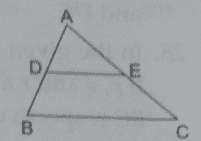Sol:

In $\bigtriangleup ADE \;\; and \;\;\bigtriangleup ABC$

$\angle ADE = \angle ABC$   ( Corresponding angles in $DE \parallel BC$ )

$\angle AED = \angle ACB$   ( Corresponding angles in $DE \parallel BC$ )

By AA similarity criterion, $\bigtriangleup ADE \sim \bigtriangleup ABC$

If two traingles are similar, then the ratio of their corresponding sides are proportional

$Therefore, \frac{AD}{AB} = \frac{AE}{AC}$

$\Rightarrow \frac{AD}{AD + DB} = \frac{AE}{AE + EC}$

$\Rightarrow \frac{x}{x + 3x + 4} = \frac{x + 3}{x + 3 + 3x + 19}$

$\Rightarrow \frac{X}{4x + 4} = \frac{x + 3}{4x + 22}$

$\Rightarrow \frac{x}{2x + 2} = \frac{x + 3}{2x + 11}$

$\Rightarrow 2x^{2} + 11x = 2x^{2} + 2x + 6x + 6$

$\Rightarrow 3x = 6$

$\Rightarrow x = 2$

Hence the value of x is 2

Q.15: A ladder 10 m long reaches the window of a house 8 m above the ground. Find the distance of the foot of the ladder from the base of the wall.

Sol: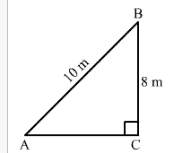Let, AB be a ladder and B is the window at 8 m above the ground C.

Now, in right triangle ABC

By using pythagoras theorem , we have

$AB^{2} = BC^{2} + CA ^{2}$

$\Rightarrow 10^{2} = 8^{2} + CA ^{2}$

$\Rightarrow CA ^{2} = 100 – 64$

$\Rightarrow CA ^{2} = 36$

$\Rightarrow CA = 6$ M

Hence, the distance of the foot of the ladder from the base of the wall is 6 m.

Q.16: Find the length of the altitude of an equilateral triangle of side 2a cm.

Sol: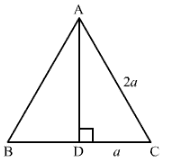We know that the altitude of an equilateral triangles bisect the side on which it stands and forms right angled triangles with the remaining sides.

Suppose ABC is an equilateral triangle having AB= BC = CA = 2a

Suppose AD is the altitude drawn from the vertex A to the side BC.

So, it will bisect the side BC

$Therefore, DC = a$

By using Pythagoras theorem, we have

$AC^{2} = CD^{2} + DA ^{2}$

span class=”latex-for-amp”>$\Rightarrow (2a)^{2} = a^{2} + DA^{2}$

$\Rightarrow DA^{2} = 4a^{2} – a^{2}$

$\Rightarrow DA^{2} = 3a^{2}$

$\Rightarrow DA = \sqrt{3 }a$

Hence, the length of the altitude of an equilateral triangle of side 2a cm is $\sqrt{3 }a$ cm

Q.17: $\bigtriangleup ABC \sim \bigtriangleup DEF$ such that ar ( $\bigtriangleup ABC$ ) = $64 \;\; cm^{2}$ and ar ( $\bigtriangleup DEF$ ) = $169 \;\; cm^{2}$. If BC = 4 c, find EF.

Sol:

We have $\bigtriangleup ABC \sim \bigtriangleup DEF$

If two triangles are similar, then the ratio of their areas to the ratio of the squares of their corresponding sides.

$Therefore, \frac{area (\bigtriangleup ABC)}{area (\bigtriangleup DEF)} = \left ( \frac{BC}{EF} \right )^{2}$

$\Rightarrow \frac{64}{169} = \left ( \frac{BC}{EF} \right )^{2}$

$\Rightarrow \left ( \frac{8}{13} \right )^{2} = \left ( \frac{4}{EF} \right )^{2}$

$\Rightarrow \left ( \frac{8}{13} \right ) = \left ( \frac{4}{EF} \right )$

$\Rightarrow EF = 6.5 \;\; cm$

Q.18: In a trapezium ABCD, it is given that $AB \parallel CD$ and $AB = 2 CD$. Its diagonals AC and BD intersect at the point O such that  ar ( $\bigtriangleup AOB$ ) = $84 \;\; cm^{2}$. Find  ar ( $\bigtriangleup COD$ ).

Sol: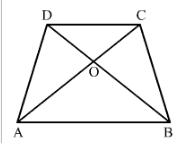In $\bigtriangleup AOB \;\; and \;\; COD$

$\angle ABO = \angle CDO$    ( Alternate angles in $AB \parallel CD$ )

$\angle AOB = \angle COD$    ( Vertically opposite angles )

If two traingles are similar, then the ratio of their areas is equal to the ratio of the squares of their corresponding sides.

$Therefore, \frac{area (\bigtriangleup AOB)}{area (\bigtriangleup COD)} = \left ( \frac{AB}{CD} \right )^{2}$

$\Rightarrow \frac{84}{area (\bigtriangleup COD)} = \left ( \frac{2. CD}{CD} \right )^{2}$

$Therefore, the area (\bigtriangleup COD) = 12 \;\; cm^{2}$

Q.19: The corresponding sides of two similar triangles are in the ratio 2:3 . If the area of the similar triangle is 48 $cm^{2}$, find the area of the larger triangle.

Sol:

If two trianges are similar , then the ratio of their areas to the ratio of the squares of their corresponding sides

$Therefore, \frac{area \;\; of \;\; the \;\; smaller \;\; traingle }{area \;\; of \;\; the \;\; larger \;\; traingle} = \left ( \frac{side \;\; of \;\; smaller \;\; traingle}{side \;\; of \;\; larger \;\; traingle} \right )^{2}$

$\Rightarrow$ The area of the larger traingle = 108 $cm^{2}$

Q.20: In an equilateral triangle with side a, prove that the area = $\frac{\sqrt{3}}{4} a^{2}$.

Sol: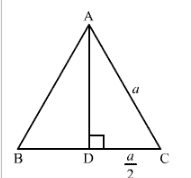We know that the altitude of an equilateral triangle bisects the side on which it stands and forms the right angled triangle with the remaining sides.

Suppose ABC is an equilateral triangle having AB = BC = CA = a.

Suppose AD is the altitude drawn from the vertex A to side BC.

So, it will bisect the side BC.

$Therefore, DC = \frac{1}{2}a$

By using Pythagoras theorem, we have

$AC^{2} = CD^{2} + DA^{2}$

$\rightarrow a^{2} = \left ( \frac{1}{2}a \right )^{2} + DA^{2}$

$\rightarrow DA^{2} = a^{2} – \frac{1}{4}a^{2}$

$\rightarrow DA^{2} = \frac{3}{4}a^{2}$

$\rightarrow DA = \frac{\sqrt{3}}{2}a$

Now, area $(\bigtriangleup ABC) = \frac{1}{2} \times BC \times AD$

$= \frac{1}{2} \times a \times \frac{\sqrt{3}}{2} a$

$= \frac{\sqrt{3}}{4}a^{2}$

Q.21: Find the length of each side of a rhombus whose diagonals are 24 cm and 10 cm long.

Sol: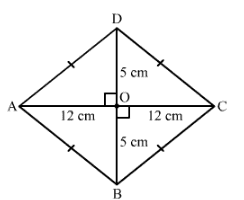Suppose ABCD is a rhombus.

We know that the diagonals of a rhombus perpendicularly bisect each other.

Therefore, angle AOB = 90, AO = 12 cm and BO = 5 cm

Now, in right triangle AOB

By using Pythagoras theorem we have

$AB^{2} = AO^{2} + BO^{2}$

$= 12^{2} + 5^{2}$

$= 144 + 25$ = 169

$Therefore, AB^{2} = 169$

$\Rightarrow AB = 13$ cm

Since, all the sides of a rhombus are equal.

Hence, AB = BC = CD = DA = 13 cm

Q.22: Two triangle DEF and GHK are such that $\angle D = 48 ^{\circ}$ and $\angle H = 57 ^{\circ}$. If $\bigtriangleup DEF \sim \bigtriangleup GHK$ then find the measure of $\angle F$.

Sol:

In two triangles are similar then the corresponding angles of the two triangles are equal.

Here, $\bigtriangleup DEF \sim \bigtriangleup GHK$

$Therefore, \angle E = \angle H = 57^{\circ}$

Now, in $\bigtriangleup DEF$

$\angle D + \angle E + \angle F = 180^{\circ}$   (Angle sum property of triangle)

$\Rightarrow \angle F = 180^{\circ} – 48^{\circ} – 57 ^{\circ} = 75 ^{\circ}$

Q.23: In the given figure, $MN \parallel BC$ and AM: MB = 1:2.  Find $\frac{area(\bigtriangleup AMN)}{area(\bigtriangleup ABC}$.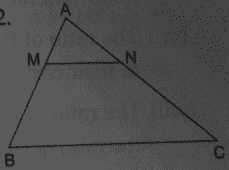Sol:

We have AM: MB = 1: 2

$\Rightarrow \frac{MB}{AM} = \frac{2}{1}$

Adding 1 to both sides, we get

$\Rightarrow \frac{MB}{AM} +1 = \frac{2}{1} + 1$

$\Rightarrow \frac{MB + AM}{AM} = \frac{2 + 1}{1}$

$\Rightarrow \frac{AB}{AM} = \frac{3}{1}$

Now, in $\bigtriangleup AMN \;\; and \;\; \bigtriangleup ABC$

$\angle AMN = \angle ABC$     ( corresponding angles in $MN \parallel BC$ )

$\angle ANM = \angle ACB$     ( corresponding angles in $MN \parallel BC$ )

By AA similarity criterion, $\bigtriangleup AMN \sim \bigtriangleup ABC$

If two triangles are similar then the ratio of their areas is equal to the ratio of the square of their corresponding sides.

$Therefore, \frac{area (\bigtriangleup AMN)}{area (\bigtriangleup ABC)}= \left ( \frac{AM}{AB} \right )^{2} = \left ( \frac{1}{3} \right )^{2} = \frac{1}{9}$

Q24: In triangle BMP and CNR it is given that PB = 5 cm, MP = 6 cm, BM = 9 cm and NR = 9 cm. If $\bigtriangleup BMP \sim \bigtriangleup CNR$, then find the perimeter of $\bigtriangleup CNR$.

Sol:

When two triangles are similar, then the ratios of the lengths of their corresponding sides are proportional.

Here, $\bigtriangleup BMP \sim \bigtriangleup CNR$

$Therefore, \frac{BM}{CN} = \frac{BP}{CR} = \frac{MP}{NR}$ ……(i)

Now, $\frac{BM}{CN} = \frac{MP}{NR}$ [ Using (i) ]

$\Rightarrow CN = \frac{BM \times NR}{MP} = \frac{9 \times 9}{6} = 13.5$ cm

$\frac{BM}{CN} = \frac{BP}{CR}$    [ Using (i) ]

$\Rightarrow CR = \frac{BP \times CN}{BM} = \frac{5 \times 13.5}{9} = 7.5$ cm

Perimeter of $\bigtriangleup CNR = CN + NR + CR = 13.5 + 9 + 7.5 = 30$ cm

Q.25: Each of equal sides of an isosceles triangle is 25 cm . Find the length of its altitude if the base is 14 cm.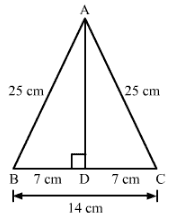Sol:

We know that the altitude drawn from the vertex opposite to the non equal side bisects the non equal side.

Suppose ABC is an isosceles triangle having sides AB and BC.

So, the altitude drawn from the vertex will bisect the opposite side. Now, In triangle ABD

By using Pythagoras theorem, we have

$AB^{2} = BC ^{2} + DA^{2}$

$\Rightarrow 25^{2} = 7 ^{2} + DA^{2}$

$\Rightarrow DA^{2} = 625 – 49$

$\Rightarrow DA^{2} = 576$

$\Rightarrow DA = 24$ cm

### Key Features of RS Aggarwal Class 10 Solutions Chapter 4 – Traingles Ex 4C (4.3)

• These solutions simplify complex topics for better understanding of the students.
• It has a collection of important questions for students to practice from each and every topic.
• The RS Aggarwal Maths Solutions is the perfect study material for revision purpose.
• It helps in improving students performance level.

#### Practise This Question

What are bio-mass fuels?